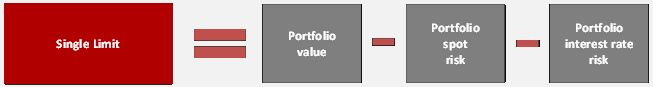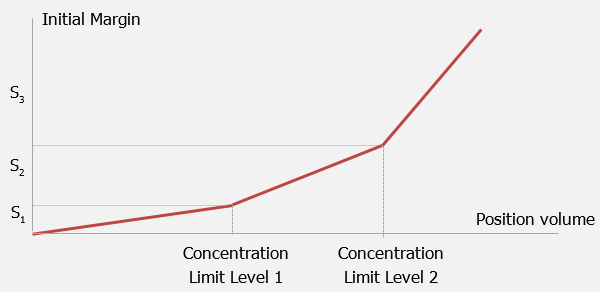Ru  /  En

# IM Calculation

The single Limit calculation is based on:

• portfolio mark-to-market
• portfolio spot risk
• portfolio interest rate risk

The single limit represents the volume of free assets which can be used as collateral. Participant's posted collateral, positions and portfolio risks are reassessed in the course of trades. Also interproduct spreads account for mitigation effect on spot risk for oppositely directed positions for assets put together in one group based on the assumption of common risk factor or high correlation

Single Limit structure can be described the following way:Principles of Single Limit Calculation

Concentration Limits for IM calculation. Margin requirements are defined in accordance to three distinct concentration levels, so more concentrated positions entail more conservative margin rates. IM depends on the position volume (net obligation, net claims and posted collateral). If the position volume is smaller than the Concentration Limit Level 1, risk rate Level 1 is applied. If the position volume is larger than the Concentration Limit Level 1 and smaller than the Concentration Limit Level 2, risk rate Level 1 is applied to the volume of Concentration Limit Level 1 and risk rate Level 2 is applied to the volume of the position exceeds Concentration Limit Level 1. If the position volume is larger than the Concentration Limit Level 2, risk rate Level 1 is applied to the volume of Concentration Limit Level 1, risk rate Level 2 is applied to the volume of difference between Concentration Limit Level 2 and Concentration Limit Level 1, and risk rate Level 3 is applied to the volume of the position exceeds Concentration Limit Level 2.Calculator

Spot risk rates

Interest risk rates